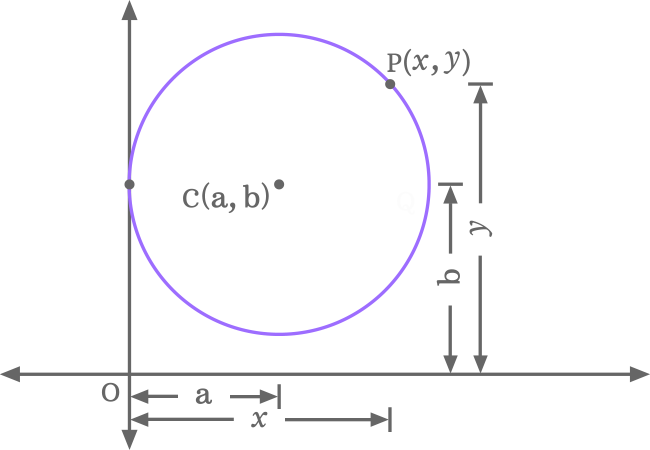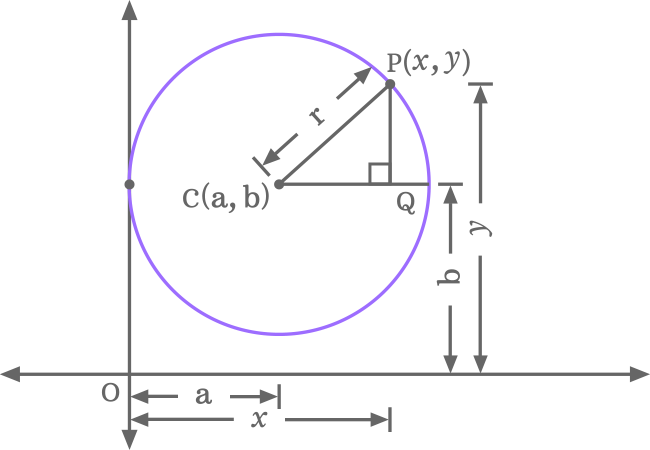# Equation of the circle when the circle touches y-axis by passing through a point

## Definition

A mathematical expression that represents a circle when the circle touches the vertical axis at a point is called equation of a circle when the circle touches $y$-axis.

In a special case, a circle can be passed through vertical $y$-axis at a point on the plane. The circle can be expressed in algebraic form equation by considering the geometrical aspects in the two dimensional space.

### Proof

The equation of a circle can be derived mathematically by using two different geometrical concepts if the circle is touched the $y$-axis by passing through a point in two dimensional Cartesian coordinate system.

1

#### According to Pythagoras Theorem

Image a circle of radius $r$ and it touches the vertical $y$-axis at a point on the plane. The touching point is at a distance of $b$ units from the origin of the two dimensional Cartesian coordinate system in the direction of $y$-axis.

The centre of the circle is at a distance of $a$ units from origin in $x$-axis point of view. The distance of centre of the circle is exactly equal to the distance of circle’s touching point in $y$-axis point of view.The distances of centre of the circle are $a$ in $x$-axis point of view and $b$ in $y$-axis point of view and they are known as $x$ and $y$ coordinates of the centre of the circle. If centre of the circle is denoted by point $C$, the centre of the circle is written as $C (a, b)$ in coordinate system.

The circle’s touching point on $y$-axis is not only a point of $y$-axis and it is one of the points on the circumference of the circle. So, the distance from circle’s touching point on $y$-axis to centre of the circle is radius of the circle. Therefore, the $x$-coordinate of the centre of the circle is equal to radius of the circle. In other words, $r = a$.

$P$ is any point on the circumference of the circle. It is at a distance of $x$ units in $x$-axis direction and $y$ units in $y$-axis from origin. Therefore, the point $P$ is denoted as $P (x, y)$ in coordinate system.

Join centre and point $P$ by a line. After that draw a line from point $C$ but it should be parallel to the $x$-axis. Similarly. Draw a line from point $P$ but it should be parallel to the $y$-axis. The lines drawn from points $C$ and $P$ intersect at a point $Q$. Thus, a right angled triangle $PCQ$ is constructed geometrically.

Find the lengths of all three sides of the $\Delta PCQ$ to start deriving the circle’s equation in mathematical form.

1. Length of opposite side ($\overline{PQ}$) is $PQ = OP \, – \, OQ = y \, – \, b$
2. Length of adjacent side ($\overline{CQ}$) is $CQ = OQ \, – \, OC = x \, – \, a$
3. Length of hypotenuse ($\overline{CP}$) is $CP = r$.

Use Pythagorean Theorem and apply it to $\Delta PCQ$ to write relation between its three sides.

${CP}^\, 2} = {CQ}^\, 2} + {PQ}^\, 2$

Substitute associated lengths of the sides in the Pythagoras theorem based expression.

$r^\, 2} = (x \,-\, a)^\, 2} + (y \,-\, b)^\, 2$

$\implies (x-a)^\, 2} + (y-b)^\, 2} = r^\, 2$

Geometrically, $r = a$ in this case. So, replace $r$ by $a$ in the equation.

$\implies (x-a)^\, 2} + (y-b)^\, 2} = a^\, 2$

It is the circle equation in terms of $x$-coordinate of the centre of the circle when the circle is touching the $y$-axis by passing through a point. Expand each square of difference based binomial to write the circle equation in expansion form.

$\implies x^\, 2} + a^\, 2} \,- 2ax + y^\, 2} + b^\, 2} \,- 2by = a^\, 2$

$\implies x^\, 2} + y^\, 2} \,- 2ax \,- 2by + a^\, 2} + b^\, 2} = a^\, 2$

$\implies x^\, 2} + y^\, 2} \,- 2ax \,- 2by + a^\, 2} + b^\, 2} \,- a^\, 2} =$

$\require{cancel} \implies x^\, 2} + y^\, 2} \,- 2ax \,- 2by + \cancel{a^\, 2}} + b^\, 2} \,- \cancel{a^\, 2}} =$

$\therefore \,\, x^\, 2} + y^\, 2} \,- 2ax \,- 2by + b^\, 2} =$

It is the expansion of the circle’s equation in terms of the $x$-coordinate of the centre of the circle if the circle is touching the vertical axis when it is passed through a point.

Now, replace $x$-coordinate of the circle $a$ by $r$ to express equation of this circle in terms of radius of the circle.

$\implies (x-r)^\, 2} + (y-b)^\, 2} = r^\, 2$

Expand each square of difference based binomial to express this circle’s equation in expansion form in terms of radius of the circle.

$\implies x^\, 2} + y^\, 2} \,- 2rx \,- 2by + b^\, 2} =$

The circle’s equation is expressed in four algebraic forms when the circle is touched the vertical axis at a point.

2

#### According to distance between two points

The distance from centre of the circle to any point on the circumference is equal to the radius of the circle. The distance between these two points can be found by using a geometrical formula and equal the distance between them to radius of the circle to derive equation of the circle when it is touching the $y$-axis.$C (a, b)$ and $P (x, y)$ are two points and the distance between them is $\sqrt{ {(x \,- a)}^\, 2} + {(y \,- b)}^\, 2}$. It is equal to $r$.

$\therefore \,\, r = \sqrt{ {(x \,- a)}^\, 2} + {(y \,- b)}^\, 2}$

$\implies r^\, 2} = {(x \,- a)}^\, 2} + {(y \,- b)}^\, 2$

$\implies {(x \,- a)}^\, 2} + {(y \,- b)}^\, 2} = r^\, 2$

The radius of the circle is also equal to the $x$-coordinate of the centre of the circle. In other words $r = a$. So, replace, $r$ by $a$ and then also replace $a$ by $r$ to obtain circle’s equation in terms of radius and $x$-coordinate of centre of the circle.

$\implies {(x \,- a)}^\, 2} + {(y \,- b)}^\, 2} = a^\, 2$

$\implies {(x \,- r)}^\, 2} + {(y \,- b)}^\, 2} = r^\, 2$

Expand square of each binomial in the expression to obtain the equation of the circle in expansion form when the circle is passed through $y$-axis at a point.

$\implies x^\, 2} + y^\, 2} \,- 2ax \,- 2by + b^\, 2} =$

$\implies x^\, 2} + y^\, 2} \,- 2rx \,- 2by + b^\, 2} =$

##### Equation

When the circle touches the $y$-axis by passing through a point The equation of a circle can be expressed in four types of algebraic form expressions if $a$ and $b$ are coordinate of the centre of the circle and $r$ is the radius of the circle.

The equation of the circle in terms of $x$-coordinate of centre of the circle.

${(x \,- a)}^\, 2} + {(y \,- b)}^\, 2} = a^\, 2$

The equation of the circle in terms of radius of the circle.

${(x \,- r)}^\, 2} + {(y \,- b)}^\, 2} = r^\, 2$

The equation of the circle in expansion form in terms of $x$-coordinate of centre of the circle.

$x^\, 2} + y^\, 2} \,- 2ax \,- 2by + b^\, 2} =$

The equation of the circle in expansion form in terms of radius of the circle.

$x^\, 2} + y^\, 2} \,- 2rx \,- 2by + b^\, 2} =$

Latest Math Topics
Latest Math Problems
Email subscription
Math Doubts is a free math tutor for helping students to learn mathematics online from basics to advanced scientific level for teachers to improve their teaching skill and for researchers to share their research projects. Know more